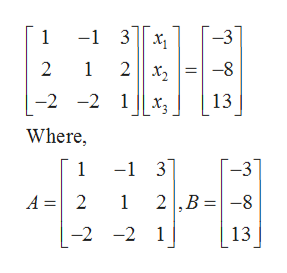# Consider the following system of equations.x1 − x2 + 3x3 = −32x1 + x2 + 2x3 = −8−2x1 − 2x2 + x3 = 13solve the system using the inverse of the cofficient matrix

Question
18 views

Consider the following system of equations.

 x1 − x2 + 3x3 = −3 2x1 + x2 + 2x3 = −8 −2x1 − 2x2 + x3 = 13

solve the system using the inverse of the cofficient matrix

check_circle

Step 1

Consider the given equations.

Step 2

Formula used:

Step 3

The augmented matrix for t...help_outlineImage Transcriptionclose1 -1 3 -3 1 2|x2 2 -8 -2 -2 1 13 x- Where, -1 3 1 A 2 1 2,B -8 -2 -2 1 13 fullscreen

### Want to see the full answer?

See Solution

#### Want to see this answer and more?

Solutions are written by subject experts who are available 24/7. Questions are typically answered within 1 hour.*

See Solution
*Response times may vary by subject and question.
Tagged in

### Matrices